Mobile QR Code1. (Department of Electronics Eng., Konkuk University, Seoul, Korea )

DFSA, RFID, Anti-collision protocols, Slotted ALOHA

## 1. Introduction

Along with wireless and non-line of sight identification capabilities of goods and people, the radio-frequency identification (RFID) technology is admitted to be an indispensable part in the Internet of Things (IoT), due to its simple architecture [1,2].

In designing RFID systems, one of the key issues is to resolve collisions resulting from simultaneous tag transmissions. The dynamic framed slotted ALOHA (DFSA) protocol is considered one of the most suitable anti-collision protocols for RFID systems, due to its performance and relatively simple implementation .

In DFSA, a frame consists of a number of time slots and the frame size is adjusted frame to frame for system efficiency. Frame size, say $L$, is determined based on number of tags, $N$, present in the interrogation zone (IZ) of the RFID reader, which is a priori unknown. The optimality of a frame size can be defined in terms of two performance metrics, i.e., $\textit{time slot utilization}$, often referred to as $\textit{normalized throughput}$, and $\textit{tag identification (ID) delay}$. In the perspective of maximizing the two metrics, the optimal frame size, say $L_{opt}$, is given by $L_{opt}=N$[4-7], and $L_{opt}=N/\ln 2\approx 1.44N$[8-12], respectively. Therefore, it is essential in DFSA to precisely estimate the number of tags for proper operation of the system.

Among several tag estimation schemes proposed thus far, $\textit{Vogt’s estimate}$ (VE) [9,10] is rated as having the best accuracy [7,13]. Nonetheless, the wide-spread use of VE is limited by the crucial drawback that its computational complexity is considerable, specially, in resource-restricted environments such as RFID systems [3,7,14].

In this study, we present a simple estimation algorithm for computing tag estimates based on VE, which turns out to be computationally very efficient. The required number of iterations is roughly $O\left(10\log _{10}N_{\max }\right)$, in contrast to $O\left(N_{\max }\right)$ in [8,9] ($N_{\max }$ represents some maximum number of tags the RFID system can accommodate). In this approach, the estimation target is system load $\lambda$ and tag population estimate $\hat{N}$ is computed from the estimated values of $\lambda$, instead of the direct approach taken in the existing method. Starting from the definition of VE, we establish a concise equation with respect to the estimate. Through computer simulations, we show that they yield identical estimates in most cases.

The rest of this paper is organized as follows. The basic system model and the existing method are described in the next section. In Section 3, our approach for tag estimation is introduced. We first derive a single equation with respect to the load estimate and then present an algorithm for the numerical solution, with some discussions on the computational complexity. In Section 4, we compare the two methods, i.e., the proposed method and VE, through computer simulations. Finally, Section 5 concludes the paper.

## 2. System model and Related Work

In this section, we first briefly describe how the DFSA protocol operates in RFID tag ID processes and then introduce the basic concepts associated with VE.

Suppose $N$ tags are positioned in the RFID reader’s IZ. The tag reading procedure proceeds on frame basis. Each DFSA frame consists of multiple time slots and the frame size, i.e. the number of time slots, $L$ is informed to the tags at the beginning of each DFSA frame. During a reading frame, each tag transmits its ID in a randomly chosen time slot, while the reader retrieves the IDs successfully transmitted by tags while gathering statistics on the number of slots filled with zero, one or multiple tag responses (a slot with multiple tag responses implies a collision within the slot). This procedure repeats frame-to-frame in the same fashion until the reader terminates the process. The overall tag ide

ntification procedure is depicted in Fig. 1. For more details, the reader is referred to [3,8,15]. Fix a DFSA frame and let random variable (RV) $X_{\mathrm{\ell }}$ denote the number of tags transmitting in $\mathrm{\ell }^{th}$ slot of the frame ($\mathrm{\ell }=1,2,\ldots ,L$). Accordingly, for each slot with either $X_{\mathrm{\ell }}=0$, $X_{\mathrm{\ell }}=1$, or $X_{\mathrm{\ell }}\geq 2$, we use the terms: $\textit{empty}$, $\textit{success}$, $\textit{collision}$, respectively. It is well known that $X_{\mathrm{\ell }}$ takes Binomial distribution, i.e.,

##### (1)
$P\left[X_{\mathrm{\ell }}=k\right]=\left(\begin{array}{l} N\\ k \end{array}\right)\left(1/L\right)^{k}\left(1-1/L\right)^{N-k},k=0,1,\ldots ,N,$

with $X_{1}+X_{2}+\cdots +X_{L}=N$ ($N$ is unknown to the reader a priori). If $E$, $S$, and $C$ denote the number of empty, success, and collision slots during the frame, respectively, RV’s $E$, $S$, and $C$ can be expressed as $E=\sum _{\mathrm{\ell }=1}^{L}1\left(X_{\mathrm{\ell }}=0\right)$, $S=\sum _{\mathrm{\ell }=1}^{L}1\left(X_{\mathrm{\ell }}=1\right)$, $E=\sum _{\mathrm{\ell }=1}^{L}1\left(X_{\mathrm{\ell }}\geq 2\right)$, respectively, where function $1\left(\cdot \right)$ denotes the indicator function, being equal to $1$ if the argument is true, and $0$, otherwise. Thus, the average number of empty, success, and collision slots during a DFSA frame, denoted by $\overline{E}$, $\overline{S}$, $\overline{C}$, respectively, can be computed as

##### (2a)
$\overline{E}=L\left(1-1/L\right)^{N}$,
##### (2b)
$\overline{S}=N\left(1-1/L\right)^{N-1},\,\,\,\mathrm{and}$
##### (2c)
$\overline{C}=L-\left(\overline{E}+\overline{S}\right).$

Suppose we have $\left(E,S,C\right)=\left(e,s,c\right)$ at the end of the read frame. Putting $p=\left(\overline{E},\overline{S},\overline{C}\right)$ and $q=\left(e,s,c\right)$, VE, $\overset{˜}{N}$ is defined by

##### (3)
$\overset{˜}{N}\triangleq arg\min _{N}\left\| p\right.-\left.q\right\|$,

where where $\parallel \cdot \parallel$ is Euclidean norm, thereby, $\parallel p-q\parallel$ representing the distance between two points $p$ and $q$ in the three dimensional non-negative real space, $\mathrm{\mathbb{R}}_{+}^{3}$ . Therefore, for observation $\left(e,s,c\right)$ and frame size $L$, $\overset{˜}{N}$ is obtained by choosing the value of $N$ ($N=1,2,\ldots$) for which $\parallel p-q\parallel$ is minimized.

It is generally accepted that the computational requirement for (3) is quite high [2,5,9]; it requires to evaluate the composite functions (2a)-(2c) for $N=1,2,\ldots ,N_{\max }\,,$ where $N_{max}$ denotes some (preset) maximum number of tags the RFID reader can accommodate, e.g., $N_{max}=500$ for $L=100.$ The computational burden can be lessened to some extent as follows. With $\left(e,s,c\right)$, we have the inequality

##### (4)
$N\geq s+2c,$

since the number of tag responses in a collision slot is greater than or equal to $2$. Combining (4) with (3), we can refine (3) by

##### (5)
$$\widetilde{N} \triangleq \arg \min _{N \in \Omega}\|p-q\|,$$

where $\Omega \triangleq \left[s+2c,N_{max}\right]$, referred to as $\textit{tag search range}$.

##### Fig. 1. Flowchart for DFSA-based tag identification procedures.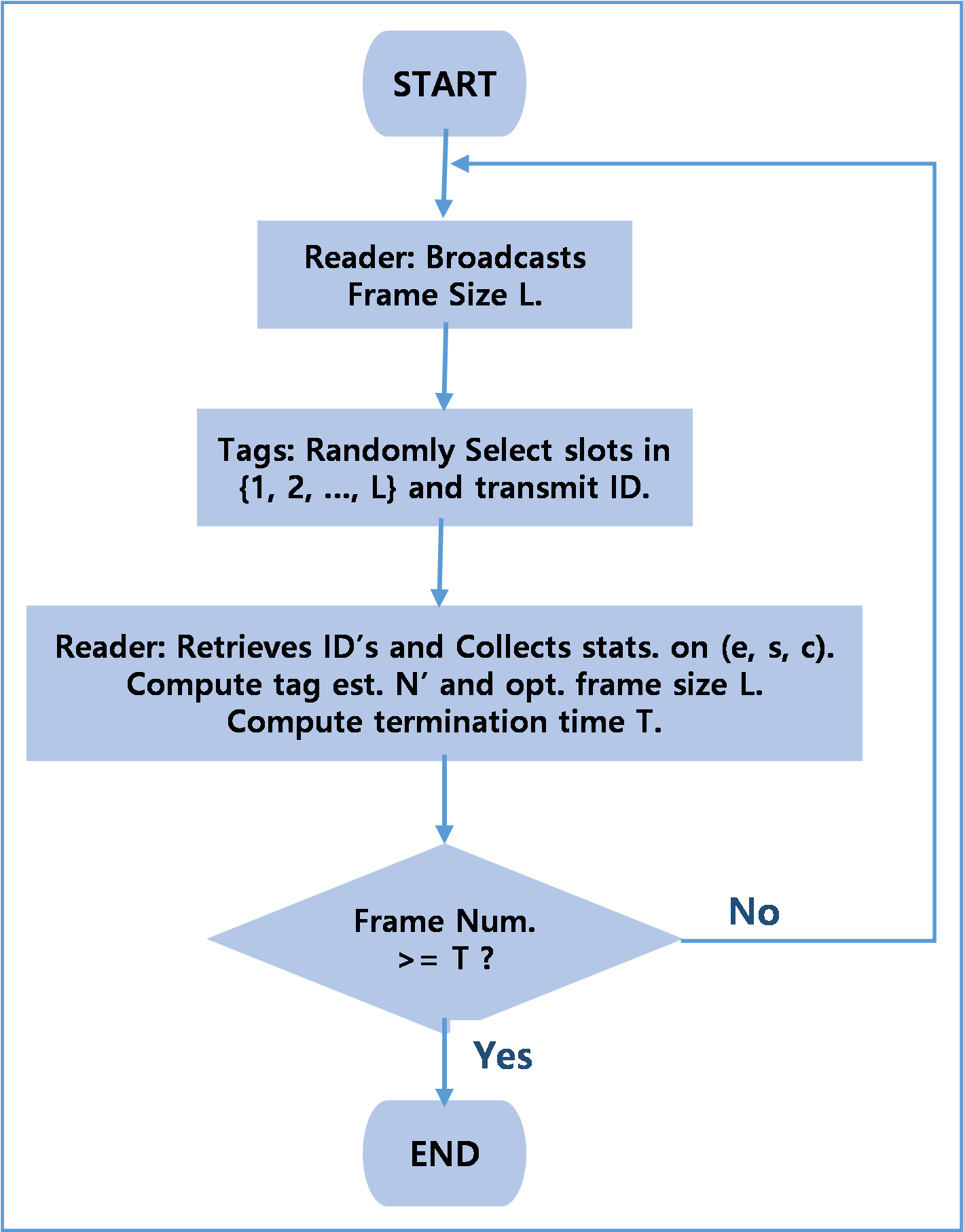## 3. The Proposed Method

If we set $\lambda \triangleq N/L$, quantity $\lambda$ represents the $\textit{system load}$, indicating the level of traffic load placed on the system. For instance, $\lambda =2$ implies the tag population is twice the frame size $L$. Note that $\lambda$ is also unknown since $N$ is unknown a priori. Instead of estimating $N$ directly, we can take two steps as follows: System load $\lambda$ is first estimated, and then estimate $\hat{N}$ is computed from the equation $\hat{N}=\left[\hat{\lambda }\times L\right]$, where function $\left[x\right]$ represents the integer closest to $x$, and $\hat{N}$ and $\hat{\lambda }$ denote the estimated values of $N$ and $\lambda$, respectively.

On the other hand, it is well known that the tag occupancy of slot $\mathrm{\ell }$, $X_{\mathrm{\ell }}$ converges in distribution to Poisson RV with parameter $\lambda$ when $N$ (or alternatively, $L$) gets large. If we fix $\lambda$ and replace $N$ in (1) by $\lambda L$, then we have

##### (6)
$P\left[X_{\mathrm{\ell }}=k\right]\rightarrow \frac{\lambda ^{k}e^{-\lambda }}{k!}\triangleq p_{k},$

as $L\rightarrow \infty ~ (k=0,1,\ldots$).

Throughout our discussion, we assume that $N$ is large and use (8) for the distribution of $X_{\mathrm{\ell }}$. Furthermore, dividing $\overline{E}$, $\overline{S}$, and $\overline{C}$ in (2) by $L$ and setting $L\rightarrow \infty$, we observe

$\overline{E}/L=\left(1-1/L\right)^{N}\rightarrow p_{0},$

$\overline{S}/L=N/L\left(1-1/L\right)^{N-1}\rightarrow p_{1},\,\,\,\mathrm{and}$

$\overline{C}/L=1-\left(\overline{E}/L+\overline{S}/L\right)\rightarrow 1-p_{0}-p_{1}.$

With the observation $\left(e,s,c\right)$ at the end of the DFSA frame, if we set $\alpha \triangleq e/L$, $\beta \triangleq s/L$, and $\gamma \triangleq c/L$, then $\alpha$, $\beta$, and $\gamma$ represent the empirical empty, success, and collision slot ratios, respectively, with $\alpha +\beta +\gamma =1$.

In the context of the definition of VE (3), we define the proposed load estimate $\hat{\lambda }$ as

##### (7)
$$\hat{\lambda} \triangleq \operatorname{argmin}_{\lambda>0}\left\|\left(p_{0}, p_{1}, p_{c}\right)-(\alpha, \beta, \gamma)\right\|,$$

where $p_{c}\triangleq P\left[X_{\mathrm{\ell }}\geq 2\right]=1-p_{0}-p_{1}$.

As is done in the previous section, dividing the inequality (4) by $L$, the estimate $\lambda$ can be bounded below as

##### (8)
$\lambda \geq \beta +2\gamma =2-\left(2\alpha +\beta \right).$

Therefore, the estimate (8) can be refined by

##### (9)
$\lambda ^{\ast }=\max \left(\hat{\lambda },2-\left(2\alpha +\beta \right)\right).$

The trajectory of point $\left(p_{0},p_{1},p_{c}\right)$, as a function of $\lambda$, is depicted in Fig. 2. Let $P$ denote a point on the trajectory for $\lambda$, i.e., $P=\left(e^{-\lambda },\lambda e^{-\lambda },1-e^{-\lambda }-\lambda e^{-\lambda }\right)$. The vector tangent to point $P,$ $\overset{\rightarrow }{r},$ is given by $\overset{\rightarrow }{r}=\frac{d}{d\lambda }\left(e^{-\lambda },\right.$ $\left.\lambda e^{-\lambda },1-e^{-\lambda }-\lambda e^{-\lambda }\right)=\left(-1,1-\lambda ,\lambda \right)e^{-\lambda }$, while the vector pointing from Q to P, $\overset{\rightarrow }{s}\triangleq \overset{\rightarrow }{QP}=\left(e^{-\lambda }-\alpha ,\lambda e^{-\lambda }-\beta ,\right.$ $\left.1-e^{-\lambda }-\lambda e^{-\lambda }-\gamma \right)$. For given observation $\left(\alpha ,\beta ,\gamma \right)$, the proposed estimate $\hat{\lambda }$ corresponds to the point on the trajectory, which is the closest to point $Q=\left(\alpha ,\beta ,\gamma \right)$. In this respect, we can say, at point $P$ corresponding to $\lambda =\hat{\lambda }$, two vectors $\overset{\rightarrow }{r}$ and $\overset{\rightarrow }{s}$ are perpendicular with each other, i.e.,

$\overset{\rightarrow }{r}\cdot \overset{\rightarrow }{s}=0,$

from which we get

##### (10)
$\left(1+2\lambda ^{2}\right)e^{-\lambda }=\left(\alpha +2\beta \right)\lambda +\alpha -\beta .$

The closed form solution of (10) is not available. We now state our approach for calculating the estimate from the Eq. (10). Let

$g\left(\lambda \right)\triangleq \left(1+2\lambda ^{2}\right)e^{-\lambda }\,\,\mathrm{and}\,\,h\left(\lambda \right)\triangleq \left(\alpha +2\beta \right)\lambda +\alpha -\beta ,$

which are depicted in Fig. 3, for $\left(\alpha ,\beta \right)=\left(0.44,0.29\right)$. Note that $h\left(\lambda \right)$ depends on the empirical slot ratios $\left(\alpha ,\beta \right)$, while $g\left(\lambda \right)$ is fixed. From the observation that $g\left(0\right)=1>h\left(0\right)=\alpha -\beta$ and $g\left(\lambda \right)\rightarrow 0$ as $\lambda \rightarrow \infty$ while $h\left(\lambda \right)$ is strictly increasing, we can tell $g\left(\lambda \right)$ crosses $h\left(\lambda \right)$ from downward at least once (it is possible that $g\left(\lambda \right)$ can cross $h\left(\lambda \right)$ more than once). We set the value of $\lambda$ at the first cross point, as our load estimate, i.e., $\hat{\lambda }$. Load estimate $\hat{\lambda }$ can be numerically computed using the iterative method stated in Algorithm 1: In line 2 of Algorithm 1, $\lambda _{\max }\triangleq N_{\max }/L$ ($\left\lceil x\right\rceil$ denotes the smallest integer greater than or equal to $x$) and, in line 5, $f\left(\lambda \right)\triangleq g\left(\lambda \right)-h\left(\lambda \right)$.

Some comments on Algorithm 1: First, Eq. (10) can have more than one root; more specifically, (10) can have one or (very rarely) three roots, but in such cases, we take the smallest one as our estimate. Second, in any execution step of Algorithm 1, if $f\left(\lambda \right)=0$ (or $f\left(\lambda +\Delta \right)=0$), the iteration terminates immediately because we have already found a root of (10), i.e., $\lambda$ (or $\lambda +\Delta$, resp.). This aspect is omitted in Algorithm 1 for simplicity. Third, the initial load search range, given by $\left[0,\lambda _{\max }\right]$ can not be replaced by $\left[2-\left(2\alpha +\beta \right),\lambda _{\max }\right]$, as was done in establishing (5), because the (first) zero-crossing point may reside in $\left(0,2-\left(2\alpha +\beta \right)\right)$. Last but most importantly, given frame size $L$, the maximum number of iterations required for Algorithm 1 can be expressed as $O\left(10\log _{10}L+\lambda _{\max }\right)$ (in line 3, $n\approx \log _{10}L$), while $O\left(N_{\max }-\left(s+2c\right)+1\right)$ iterations are required, with the narrowed tag search range $\Omega$ for VE stated in (5). For instance, in case of $L=100$ and $N_{max}=500$, roughly calculating, 25 iterations are needed for Algorithm 1, in contrast to 418 iterations for VE (assuming $s=29,c=27$).

##### Fig. 2. Trajectory of point $\left(p_{0},p_{1},p_{c}\right)$ in $\mathrm{\mathbb{R}}_{+}^{3}$, as a function of system load $\lambda$($0.4\leq \lambda \leq 1.6$), with vector $\overset{\rightarrow }{r}$, tangent to point P and vector $\overset{\rightarrow }{s}$, pointing from Q to P.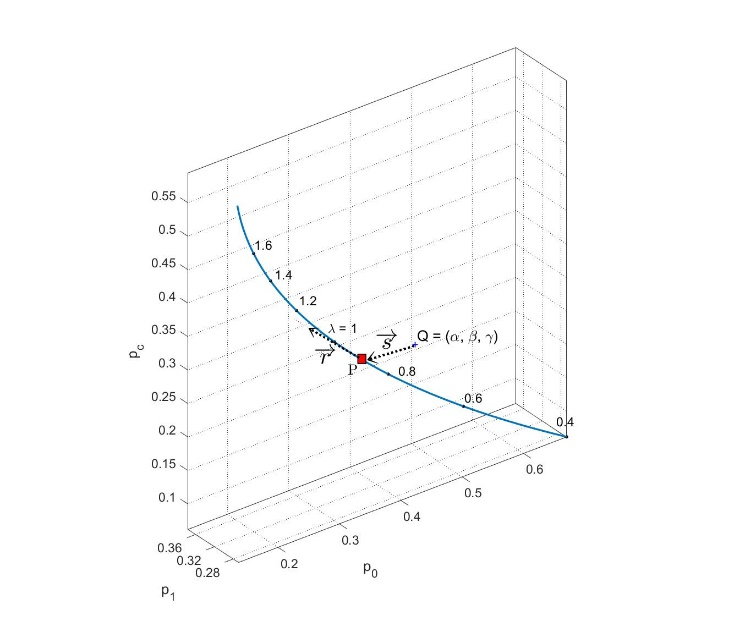##### Fig. 3. Functions $g\left(\lambda \right)$ and $h\left(\lambda \right)$ for $\left(\alpha ,\beta \right)=$ $\left(0.44,0.29\right)$.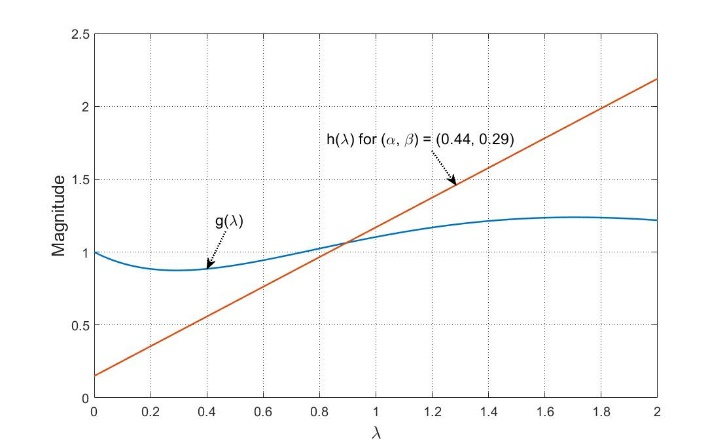##### Fig. 4. Comparison of VE and the proposed method ($N=200$, $L=200$, $\lambda =1$).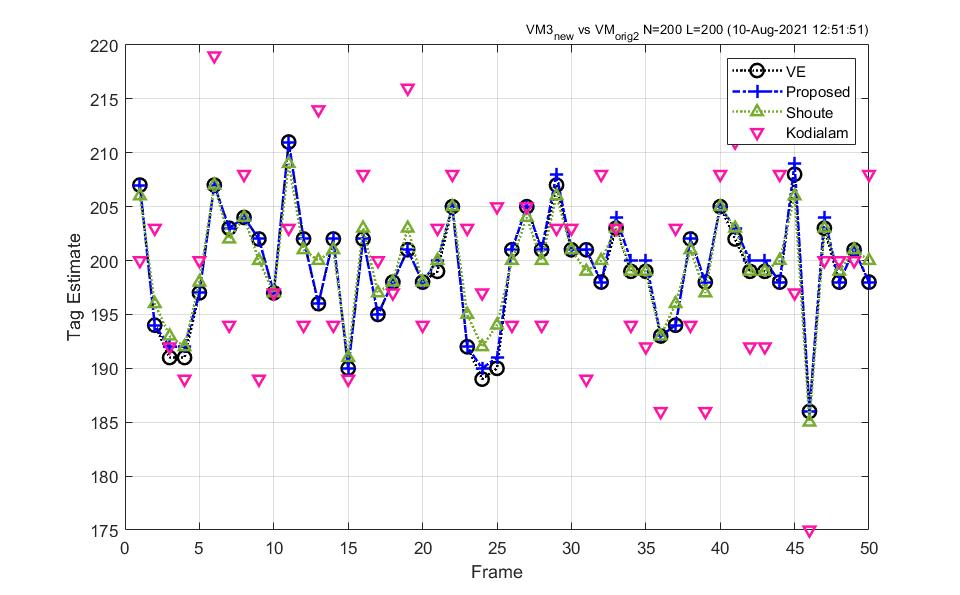##### Fig. 5. Comparison of VE and the proposed method ($N=200$, $L=100$, $\lambda =2$).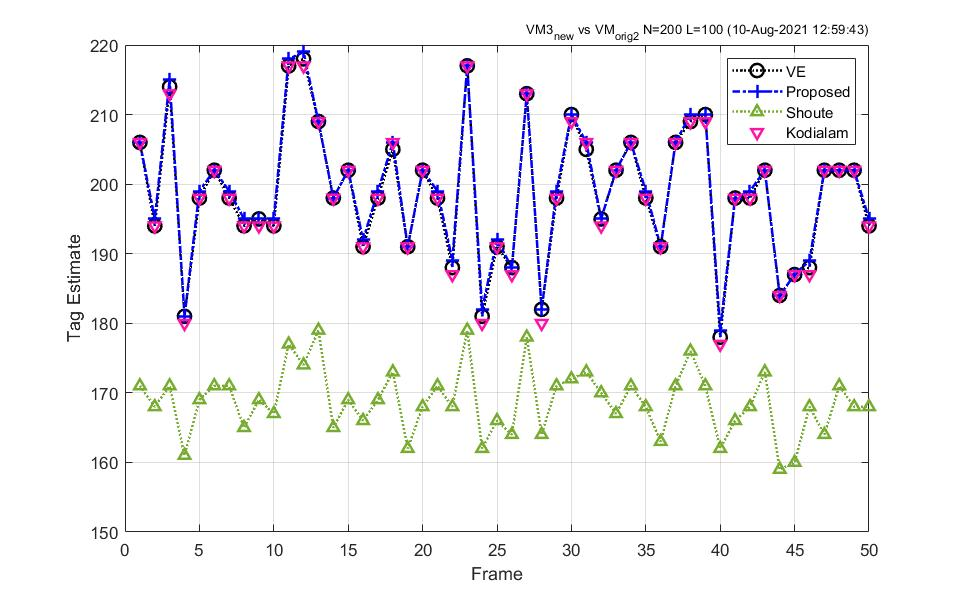##### Fig. 6. Comparison of VE and the proposed method ($N=100$, $L=200$, $\lambda =0.5$).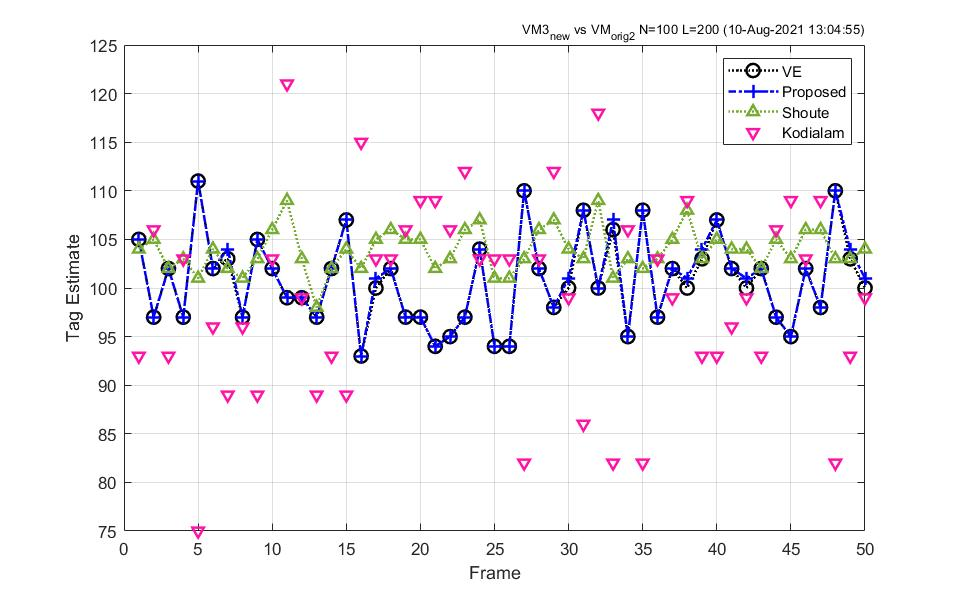## 4. Numerical Comparison Results

Figs. 4-6 compare tag estimates obtained using the proposed method (9) with the ones by VE (5), Shoute , and Kodialam’s estimates , for system load $\lambda =1,2,0.5$, where $\left(N,L\right)=\left(200,200\right),\left(200,100\right)$, and $\left(100,200\right)$, respectively. In each experiment, 50 frames of tag read procedures have been executed. The three experiments show that the two estimates are almost identical. Even for some discrepancies, the difference is insignificant as 1, mainly due to the integer rounding step: $\hat{N}=\left[\lambda ^{\mathrm{*}}\times L\right]$.

Next, we evaluated the performance of the aforementioned methods, i.e., the proposed method, VE, Shoute, and Kodialam’s estimates, in terms of normalized root-mean-square error (nRMSE) of estimates, $\varepsilon _{$\textit{nRMSE}$},$ which is defined, for $T$ sample estimates $\left\{\hat{N}_{t},t=1,2,\ldots T\right\}$, as

##### (11)
$\varepsilon _{nRMSE}\triangleq 1/N\sqrt{1/T\sum _{t=1}^{T}\left(\hat{N}_{t}-N\right)^{2}}.$

The quantity, $\varepsilon _{nRMSE}$, measures how far on average the estimate $\hat{N}$ deviates from the real value $N$. Fig. 7 depicts the comparison results in terms of nRMSE for three cases, i.e., $\lambda =1,2,0.5$ (Table 1 summarizes the nRMSE values for three cases), and shows how the nRMSE varies as $N$ increases. It can be seen that the nRMSE values of the two methods are almost identical for the three cases. The slight discrepancies found for $N=50$, however, are mainly due to the poisson approximation (for large $N$). It is also noteworthy that the estimation errors decrease as $N$ grows large. This phenomenon can be explained by the tendency that the empirical slot ratios $\left(\alpha ,\beta ,\gamma \right)$ slowly converge to $\left(p_{0},p_{1},p_{c}\right)$ as $N$ gets larger, coupled with the fact that VE is based on Chebyshev’s inequality .

##### Table 1. Comparisons in terms of nRMSE.
 λ 0.5 1 1.5 N VE Prop. VE Prop. VE Prop. 50 0.0572 0.0614 0.0563 0.0571 0.0860 0.0877 100 0.0447 0.0455 0.0387 0.0387 0.0590 0.0593 200 0.0337 0.0340 0.0274 0.0274 0.0421 0.0422 300 0.0285 0.0286 0.0223 0.0223 0.0340 0.0340 400 0.0252 0.0253 0.0192 0.0192 0.0294 0.0295 500 0.0229 0.0230 0.0173 0.0173 0.0262 0.0262 600 0.0214 0.0215 0.0160 0.0160 0.0241 0.0242 700 0.0201 0.0201 0.0147 0.0147 0.0223 0.0223 800 0.0187 0.0188 0.0136 0.0136 0.0208 0.0208 900 0.0179 0.0179 0.0128 0.0128 0.0196 0.0196 1000 0.0171 0.0171 0.0121 0.0121 0.0184 0.0184
##### Fig. 7. Comparison of VE and the proposed method in terms of nRMSE of estimates.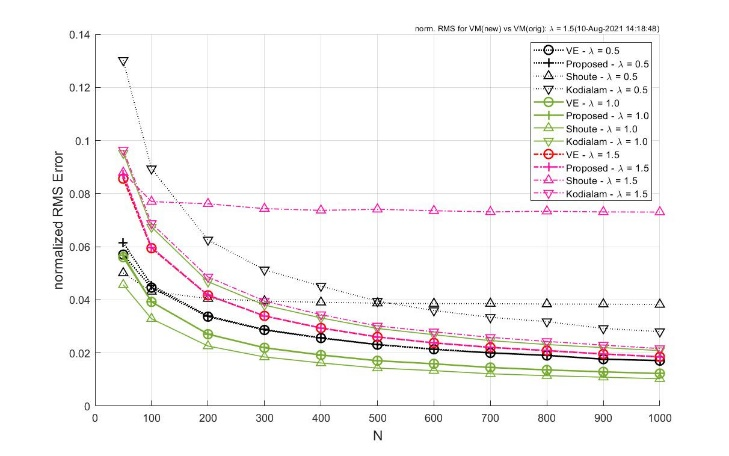## 5. Conclusion

In this paper, we presented an efficient approach for computing population estimates in DFSA-based RFID systems. In this method, the estimate of system load, $\lambda ^{\mathrm{*}}$, is computed first and population estimate $\hat{N}$ is obtained from equation $\hat{N}=\left[\lambda ^{\mathrm{*}}\times L\right]$, instead of the direct approach in the existing method. Starting from the definition of VE, we derived a concise equation with respect to the load estimate. For a numerical solution of the equation, we presented a load estimation algorithm for which the computational complexity is roughly $O\left(10\log _{10}N_{\max }\right)$, compared to $O\left(N_{\max }\right)$ for the existing method ($N_{\max }$ denoting the maximum possible number of tags). Through computer simulations, we showed that two approaches (i.e., VE and the proposed method) produce consistent results in most cases.

### REFERENCES

1
Mbacke A. A., Mitton N., 2018, ,A Survey of RFID Readers Anticollision Protocols, IEEE Journal of Radio Frequency Identification, Vol. 2, No. 1, pp. 38-482
Edwards C., 2014, RFID tags along with the Internet of Things, Eng. Technol. Mag. 9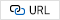3
Klair D., Chin K.-W., 2010, ,A Survey and Tutorial of RFID Anti-Collision Protocols, IEEE Communications Surveys & Tutorials, Vol. 12, No. 3, pp. 400-4214
Chen W., Lin G., 2006, An Efficient Anti-Collision Method for Tag Identification in a RFID System, IEICE-Transactions on Communications, E89-B No. 12, pp. 3386-33925
Floerkemeier C., 2006, Transmission control scheme for fast RFID object identification, 4th Annual Intl. Conference on Pervasive Computing and Communications Workshops6
Bueno-Delgado M. V., Vales-Alonso J., 2011, On the optimal frame-length configuration on real passive RFID systems, Journal of Network and Computer Applications, Vol. 34, pp. 864-8767
Zanella A., 2012, Estimating Collision Set Size in Framed Slotted Aloha Wireless Networks and RFID Systems, IEEE Communications Letters, Vol. 16, No. 3, pp. 300-3038
Zhen B., Kobayashi M., Shimizui M., 2005, Framed Aloha for multiple RFID objects identification, IEICE-Transactions on Communications, E88-B, pp. 991-9999
Vogt H., 2002, Efficient object identification with passive RFID tags, International Conference on Pervasive Computing (PerCom)10
Vogt H., 2002, Multiple object identification with passive RFID tags, IEEE Intl. Conference on Man and Cybernetics11
Prodanoff Z., 2010, Optimal frame size analysis for framed slotted ALOHA based RFID networks, Comput. Commun., Vol. 33, pp. 648-65312
Prodanoff Z., 2010, Optimal frame size analysis for framed slotted ALOHA based RFID networks, Comput. Commun., Vol. 33, pp. 648-65313
Klair D., Chin K.-W., Raad R., 2007, On the Accuracy of Tag Estimation Functions, Sixth IEEE International Symposium on Communications and Information Technologies, Sydney, Australia14
Wu H., Zeng Y., 2010, Bayesian tag estimate and optimal frame length for anti-collision Aloha RFID system, IEEE Trans. Automation Sci. and Engineer, Vol. 7, No. 4, pp. 963-96915
Cmiljanic N., Landaluce H., Perallos A., 2018, A Comparison of RFID Anti-Collision Protocols for Tag Identification, Applied Sciences 8, No. 816
Schoute F. C., 1983, Dynamic frame length ALOHA, IEEE Trans. Commun., Vol. COM-31, No. 4, pp. 565-56817
Kodialam M., Nandagopal T., pp 322-333, Fast and reliable estimation schemes in RFID systems, Proc. 2006 Annual International Conference on Mobile Computing and Networking## Author

Young-Beom Kim is a Professor of Electronics Eng. Dept. at Konkuk Univ., Seoul, Korea. He received his B.S. and M.S. degrees in Electronics Eng. from Seoul National University, Korea, in 1984 and 1986, respectively and his Ph.D. in Electrical Eng. from University of Maryland, College Park, MD, USA in 1996. His research interests include wireless networks, stochastic problems in communication systems, next generation Internet architecture, and fast packet switching systems.